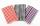# Subtracting + Mathematical Olympiad - math problems

#### Number of problems found: 1

• WipesThe mummy wiped out the square wipes and the veil was next to each other on the cord stretched out between the two trees. She used a cord of 7.5 meters in length, requiring about 8 dm on each side of the trunk. All wipes are 45 cm wide. Among the wipes an

We apologize, but in this category are not a lot of examples.
Do you have an interesting mathematical word problem that you can't solve it? Submit a math problem, and we can try to solve it.

We will send a solution to your e-mail address. Solved examples are also published here. Please enter the e-mail correctly and check whether you don't have a full mailbox.

Please do not submit problems from current active competitions such as Mathematical Olympiad, correspondence seminars etc...

Subtracting Problems. Mathematical Olympiad - math word problems.